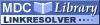Search
Browse
Statistics
Feeds

# A linear memory algorithm for Baum-Welch trainingPDF - Requires a PDF viewer such as GSview, Xpdf or Adobe Acrobat Reader 232kBItem Type: Article A linear memory algorithm for Baum-Welch training Miklos, I. and Meyer, I.M. Background: Baum-Welch training is an expectation-maximisation algorithm for training the emission and transition probabilities of hidden Markov models in a fully automated way. It can be employed as long as a training set of annotated sequences is known, and provides a rigorous way to derive parameter values which are guaranteed to be at least locally optimal. For complex hidden Markov models such as pair hidden Markov models and very long training sequences, even the most efficient algorithms for Baum-Welch training are currently too memory-consuming. This has so far effectively prevented the automatic parameter training of hidden Markov models that are currently used for biological sequence analyses. Results: We introduce the first linear space algorithm for Baum-Welch training. For a hidden Markov model with M states, T free transition and E free emission parameters, and an input sequence of length L, our new algorithm requires O(M) memory and O(LMT max (T + E)) time for one Baum-Welch iteration, where T max is the maximum number of states that any state is connected to. The most memory efficient algorithm until now was the checkpointing algorithm with O(log(L)M) memory and O(log(L)LMT (max) ) time requirement. Our novel algorithm thus renders the memory requirement completely independent of the length of the training sequences. More generally, for an n-hidden Markov model and n input sequences of length L, the memory requirement of O(log(L)L (n-1) M) is reduced to O(L (n-1) M) memory while the running time is changed from O(log(L)L (n) MT max + L (n) (T + E)) to O(L (n) MT (max) (T + E)). An added advantage of our new algorithm is that a reduced time requirement can be traded for an increased memory requirement and vice versa, such that for any c ∈ {1, ..., (T + E)}, a time requirement of L (n) MT (max) c incurs a memory requirement of L (n-1) M(T + E - c). Conclusion: For the large class of hidden Markov models used for example in gene prediction, whose number of states does not scale with the length of the input sequence, our novel algorithm can thus be both faster and more memory-efficient than any of the existing algorithms. Algorithms, Statistical Data Interpretation, Markov Chains, Mathematical Computing, Statistical Models, Sequence Alignment BMC Bioinformatics 1471-2105 BioMed Central 6 231 19 September 2005 https://doi.org/10.1186/1471-2105-6-231 View item in PubMed

Repository Staff Only: item control page